Plot combined clusterings results: classifications corresponding to Mclust/BIC and to the hierarchically combined classes, "entropy plots" to help to select a number of classes, and the tree structure obtained from combining mixture components.

# S3 method for clustCombi
plot(x, what = c("classification", "entropy", "tree"), ...)

## Arguments

x

Object returned by clustCombi function.

what

Type of plot.

...

Other arguments to be passed to other functions: combiPlot, entPlot, combiTree. Please see the corresponding documentations.

## Value

Classifications are plotted with combiPlot, which relies on the Mclust plot functions. Entropy plots are plotted with entPlot and may help to select a number of classes: please see the article cited in the references. Tree plots are produced by combiTree and graph the tree structure implied by the clusters combining process.

## Author

J.-P. Baudry, A. E. Raftery, L. Scrucca

combiPlot, entPlot, combiTree, clustCombi.

## Examples

# \donttest{
data(Baudry_etal_2010_JCGS_examples)

## 1D Example
output <- clustCombi(data = Test1D, G=1:15)

# plots the hierarchy of combined solutions, then some "entropy plots" which
# may help one to select the number of classes (please see the article cited
# in the references)
plot(output)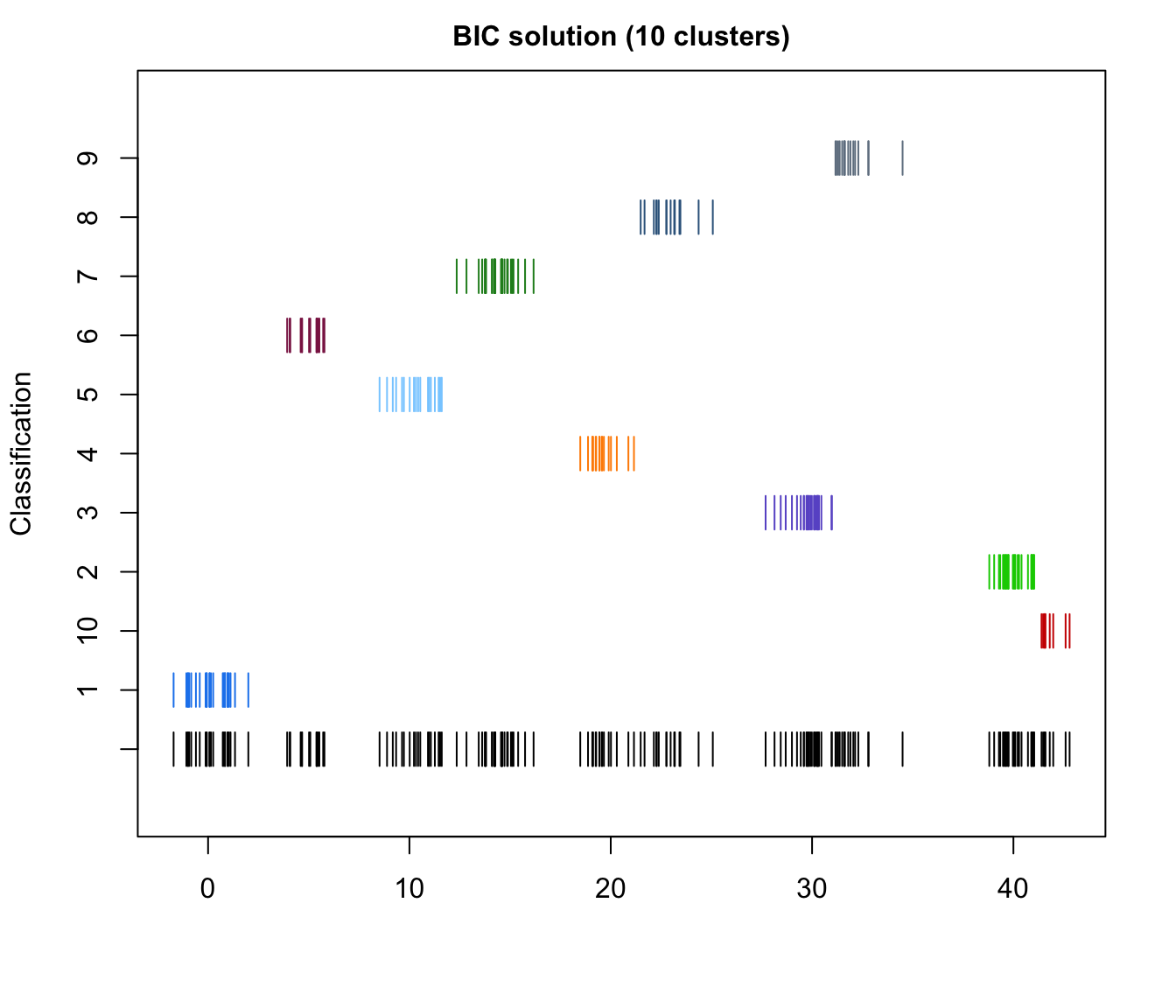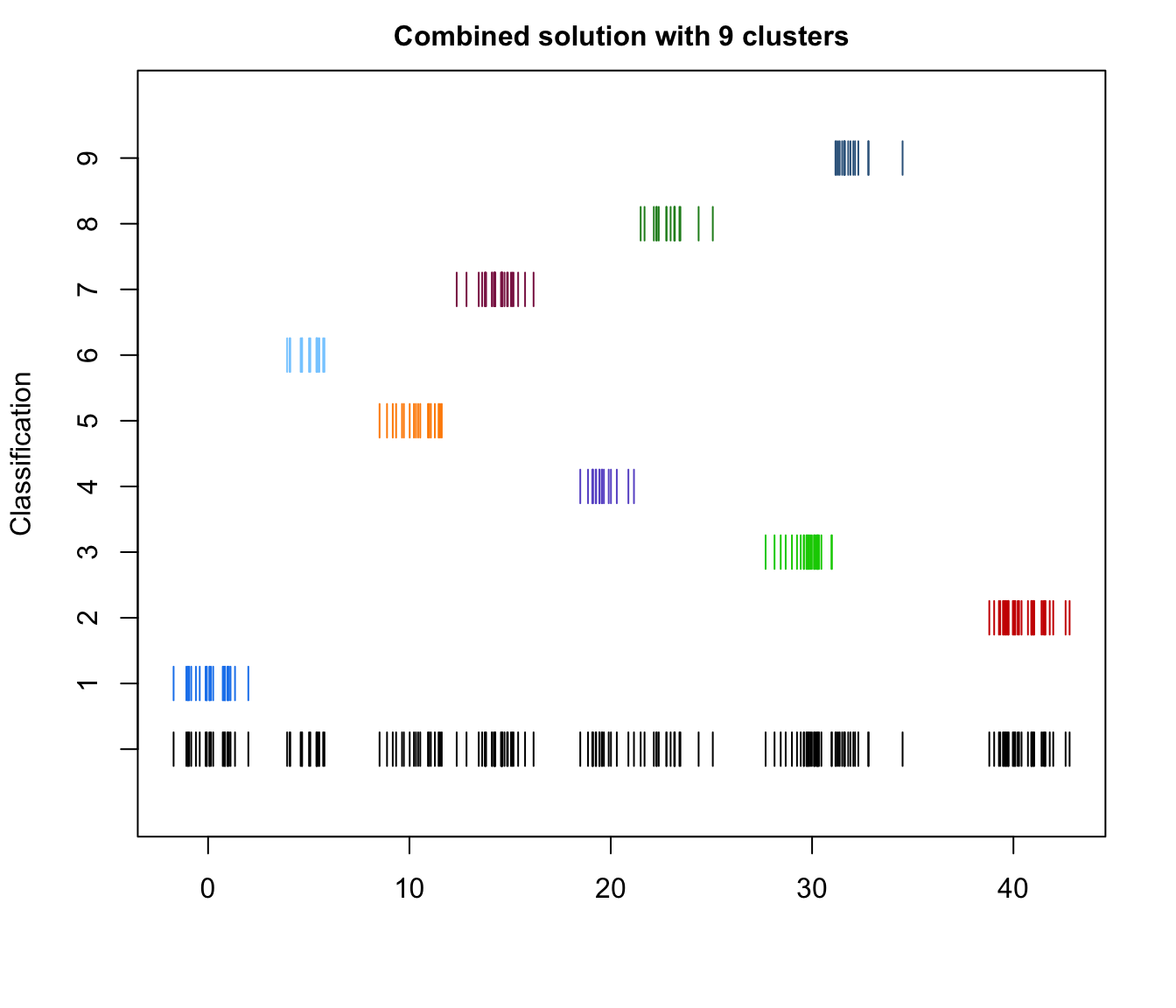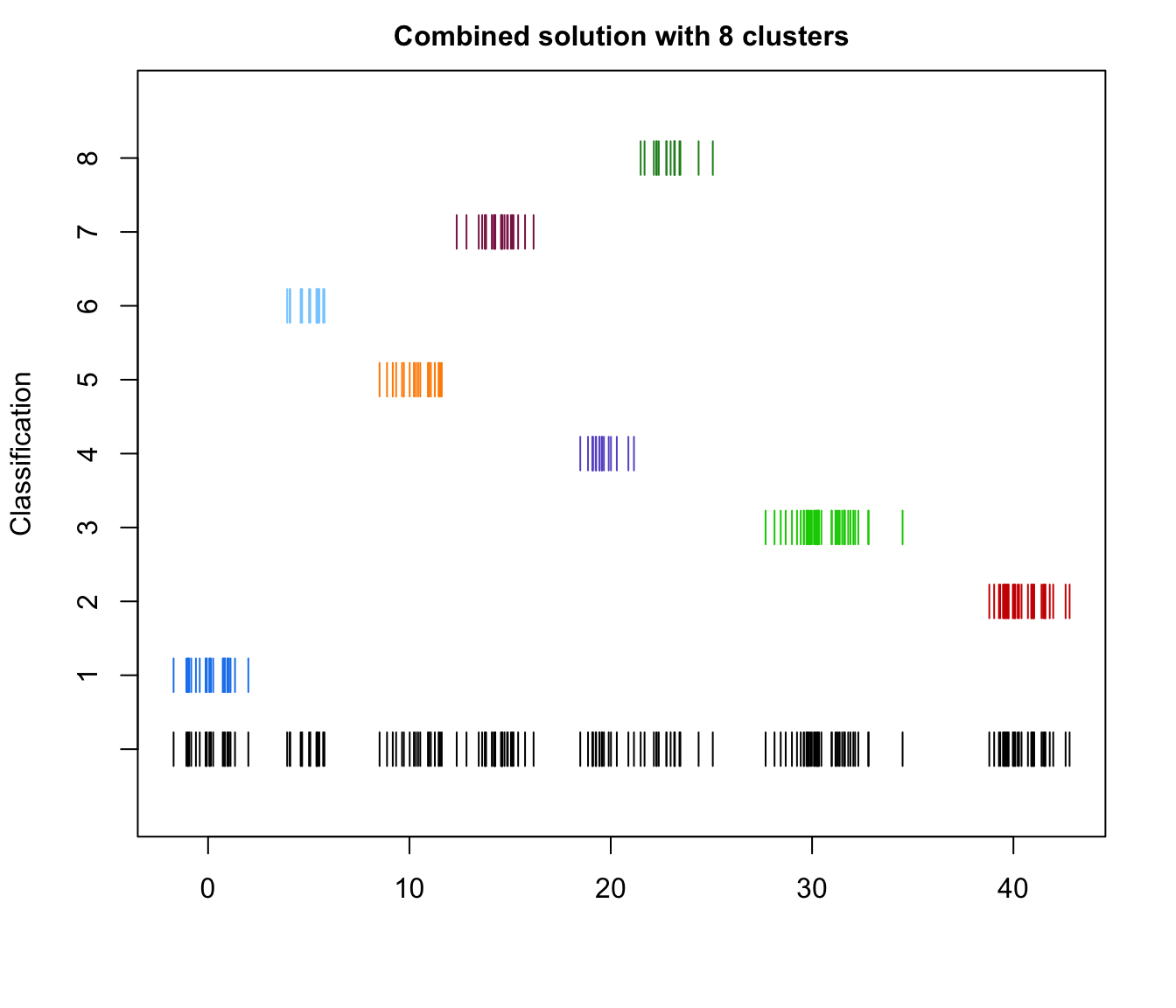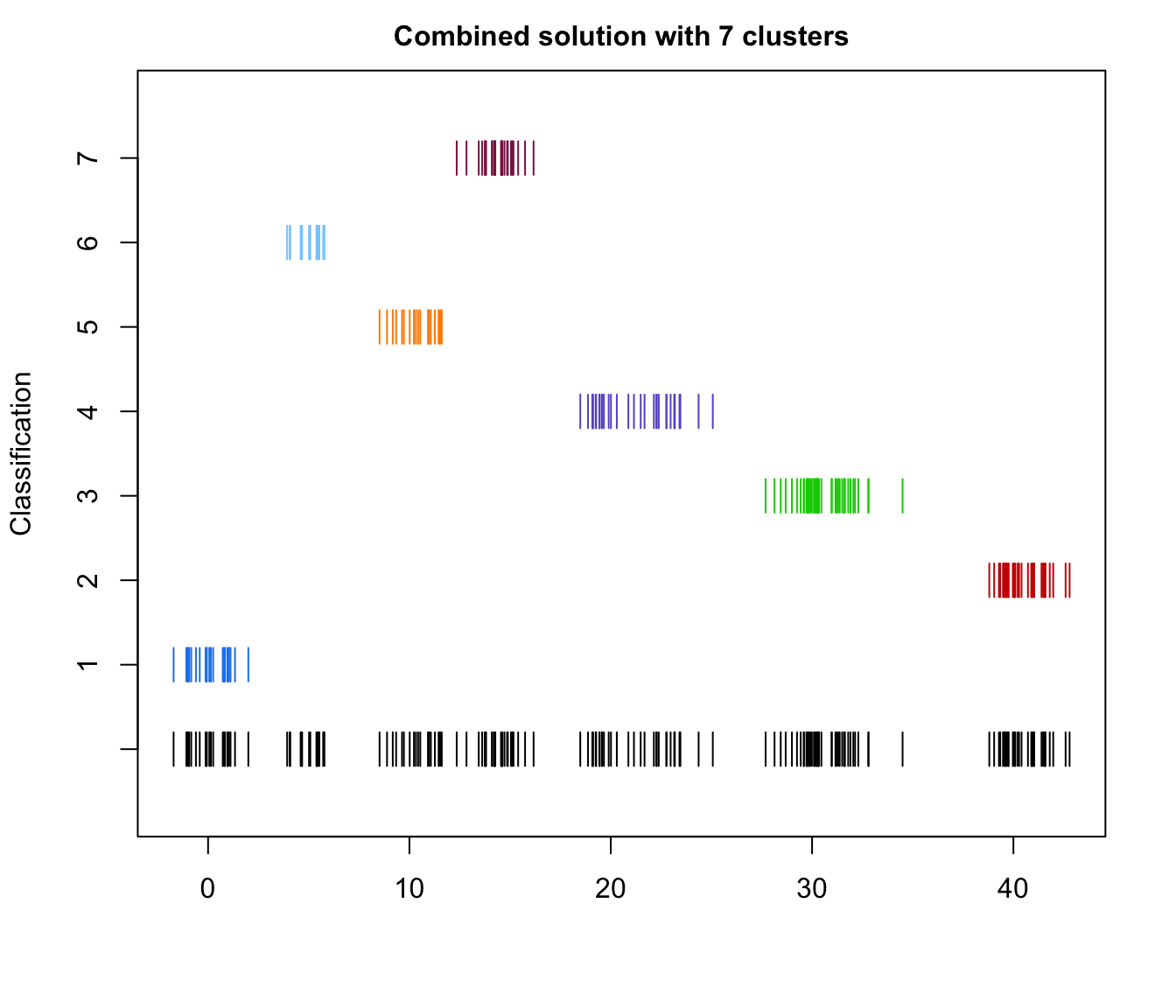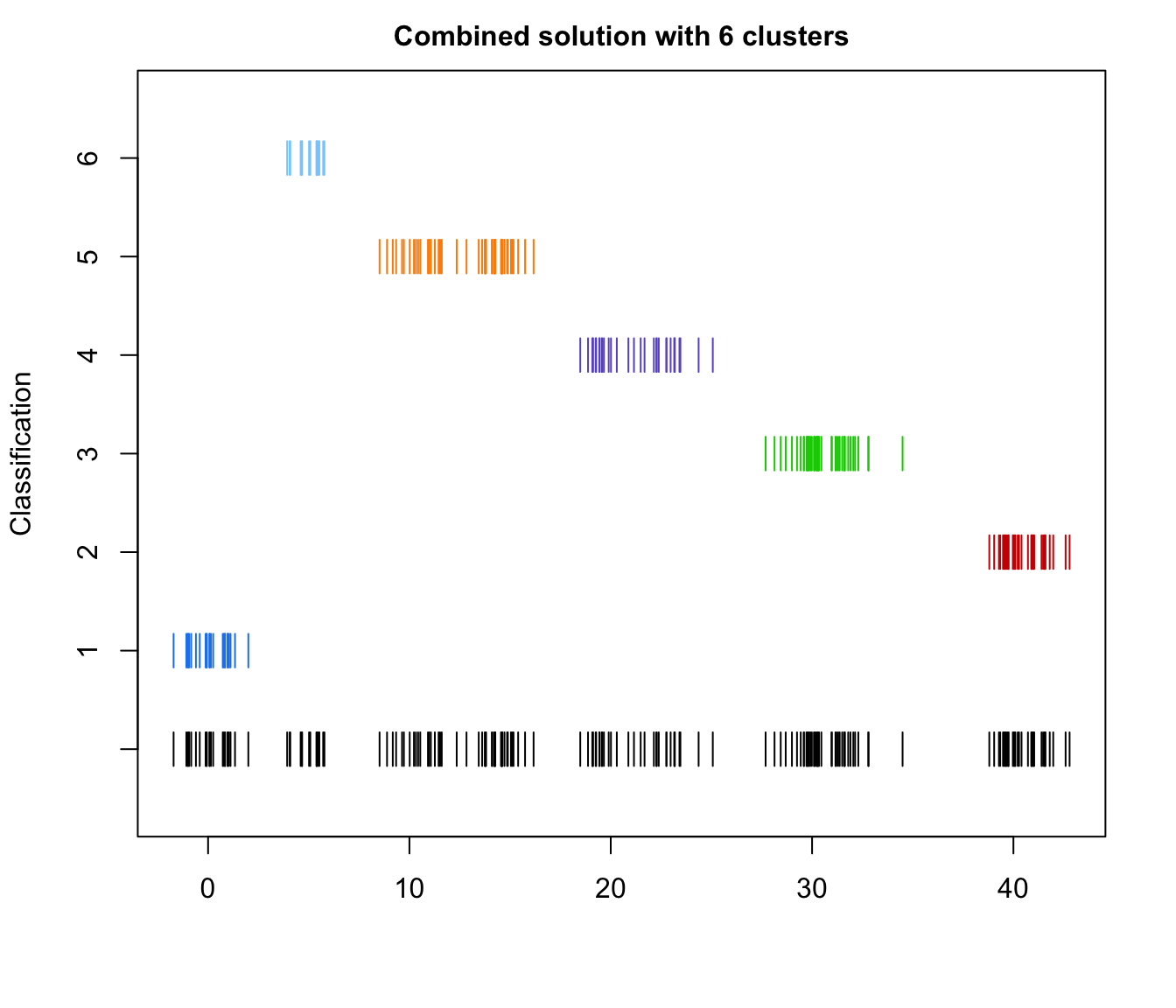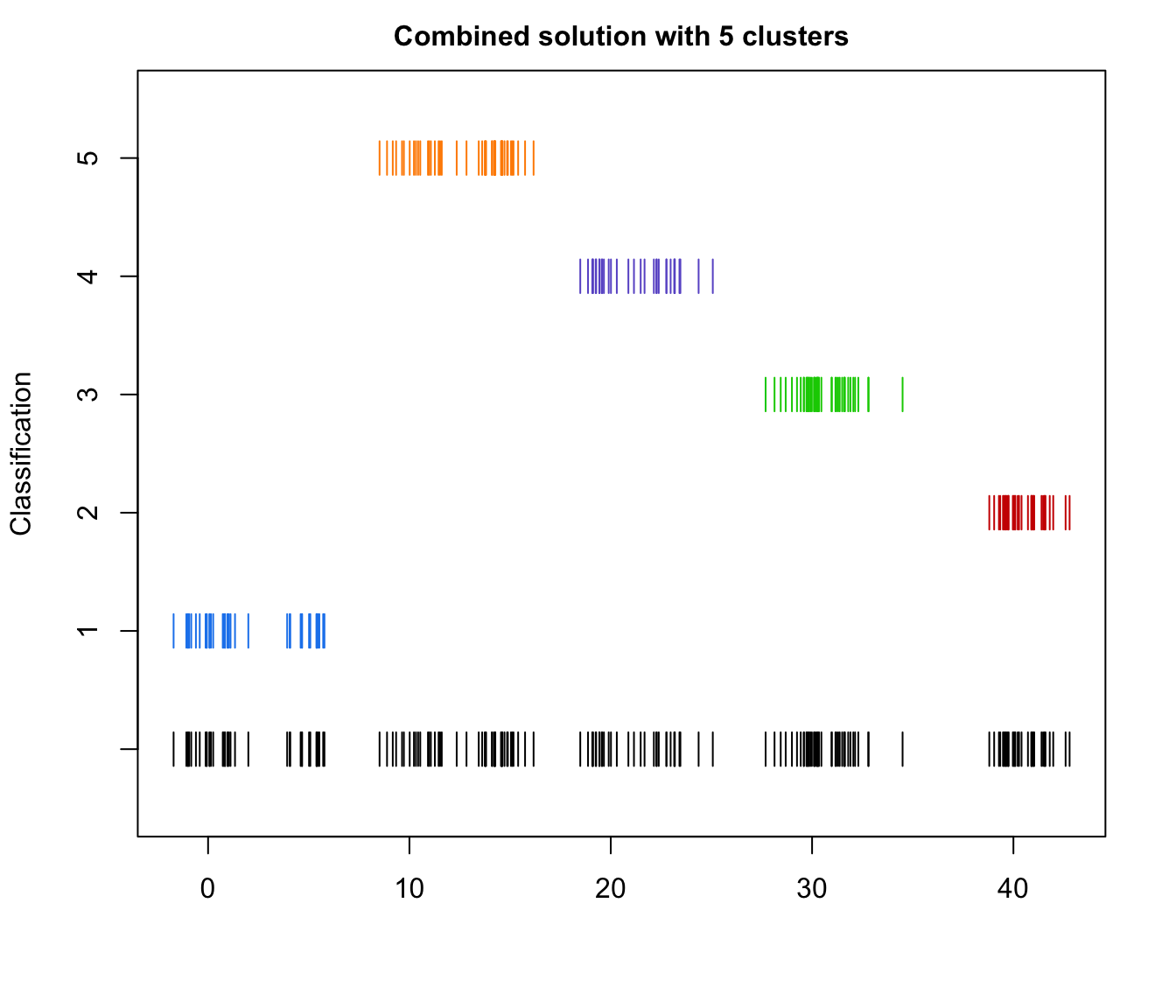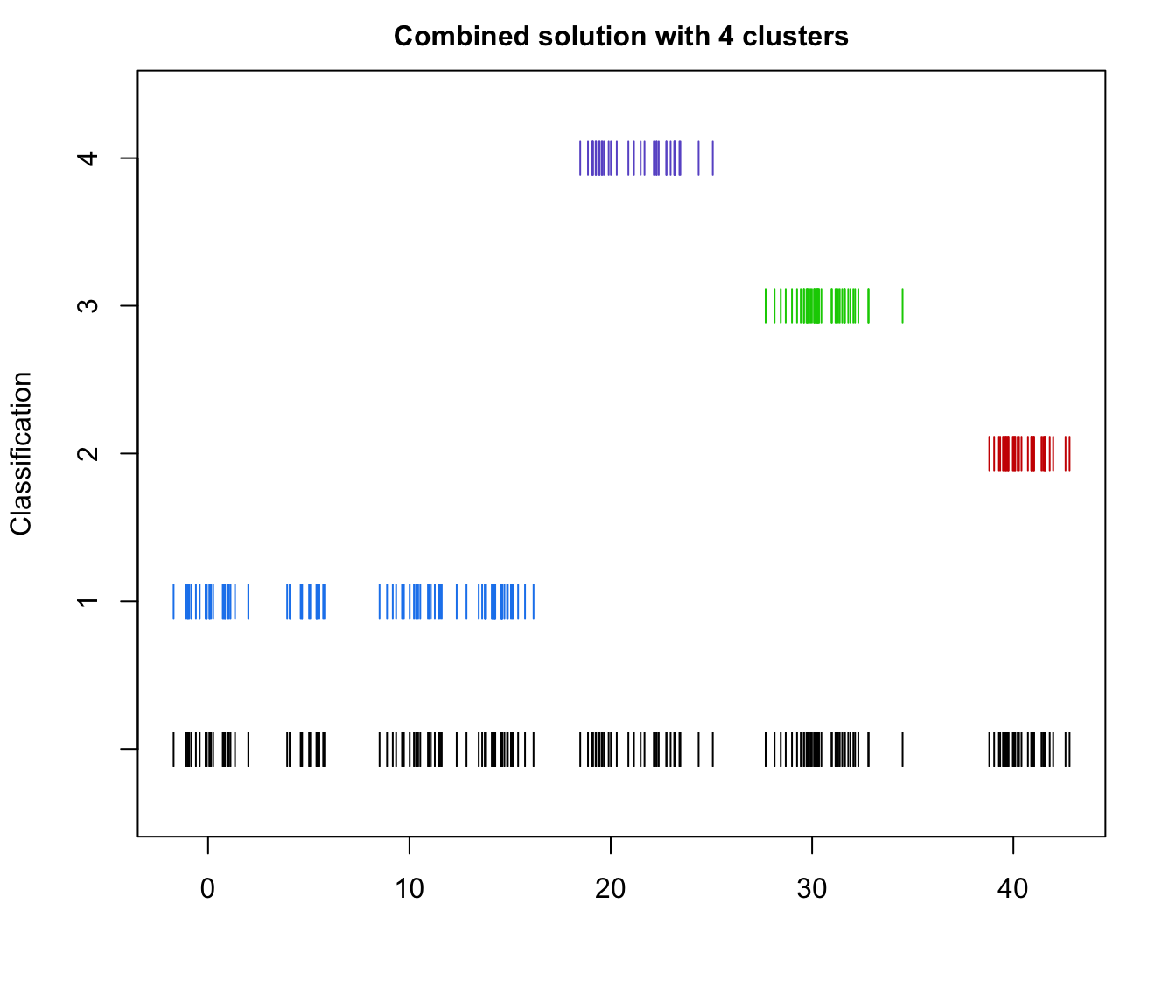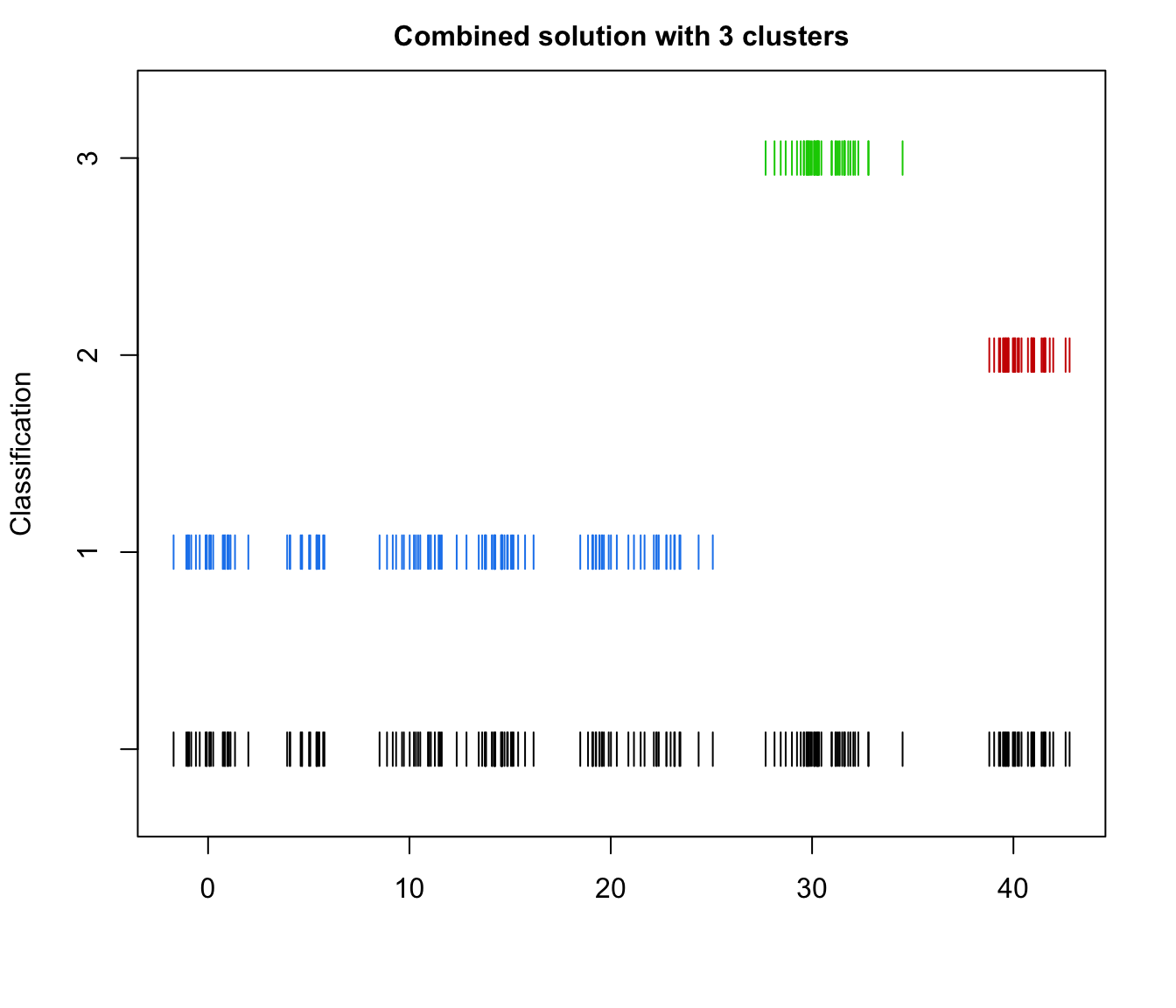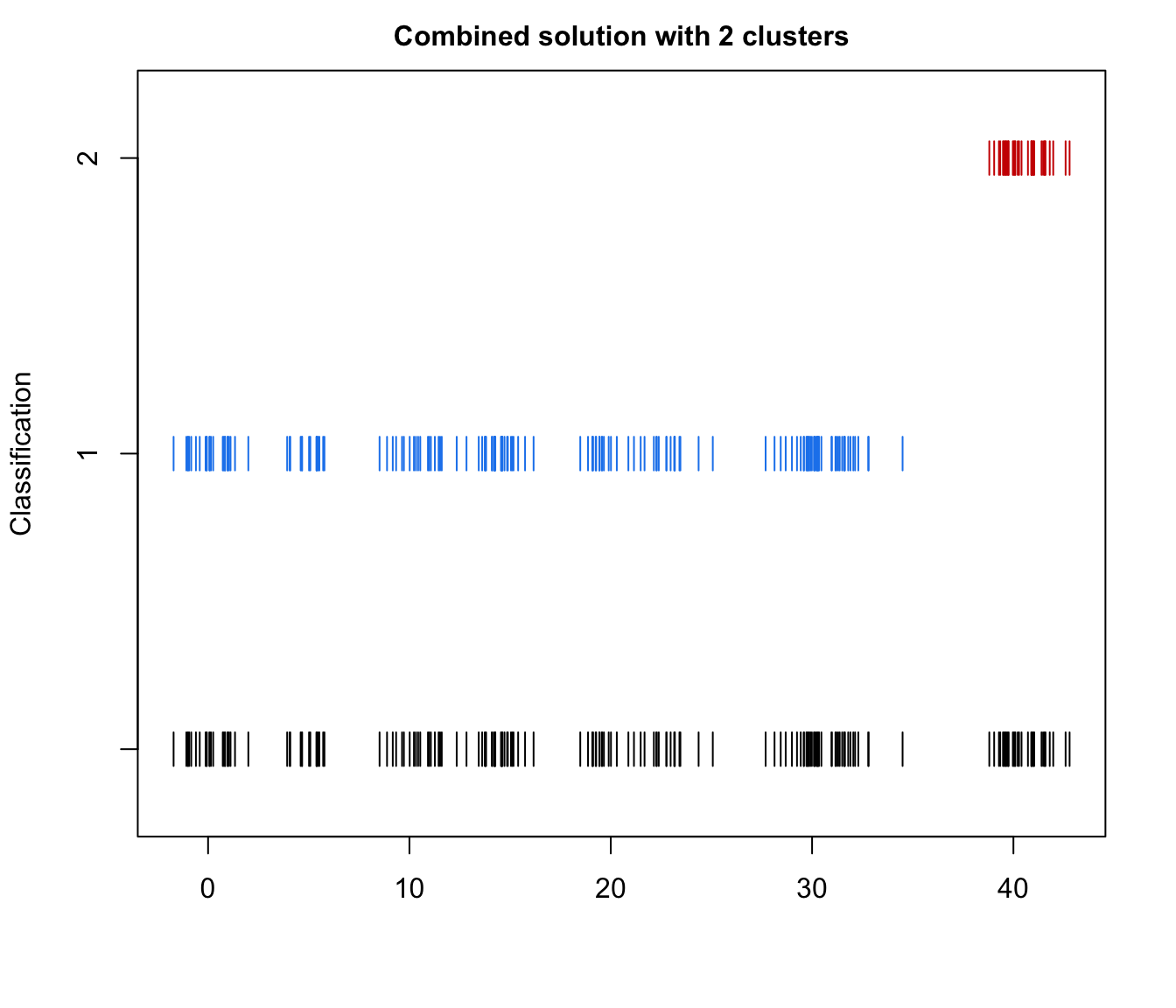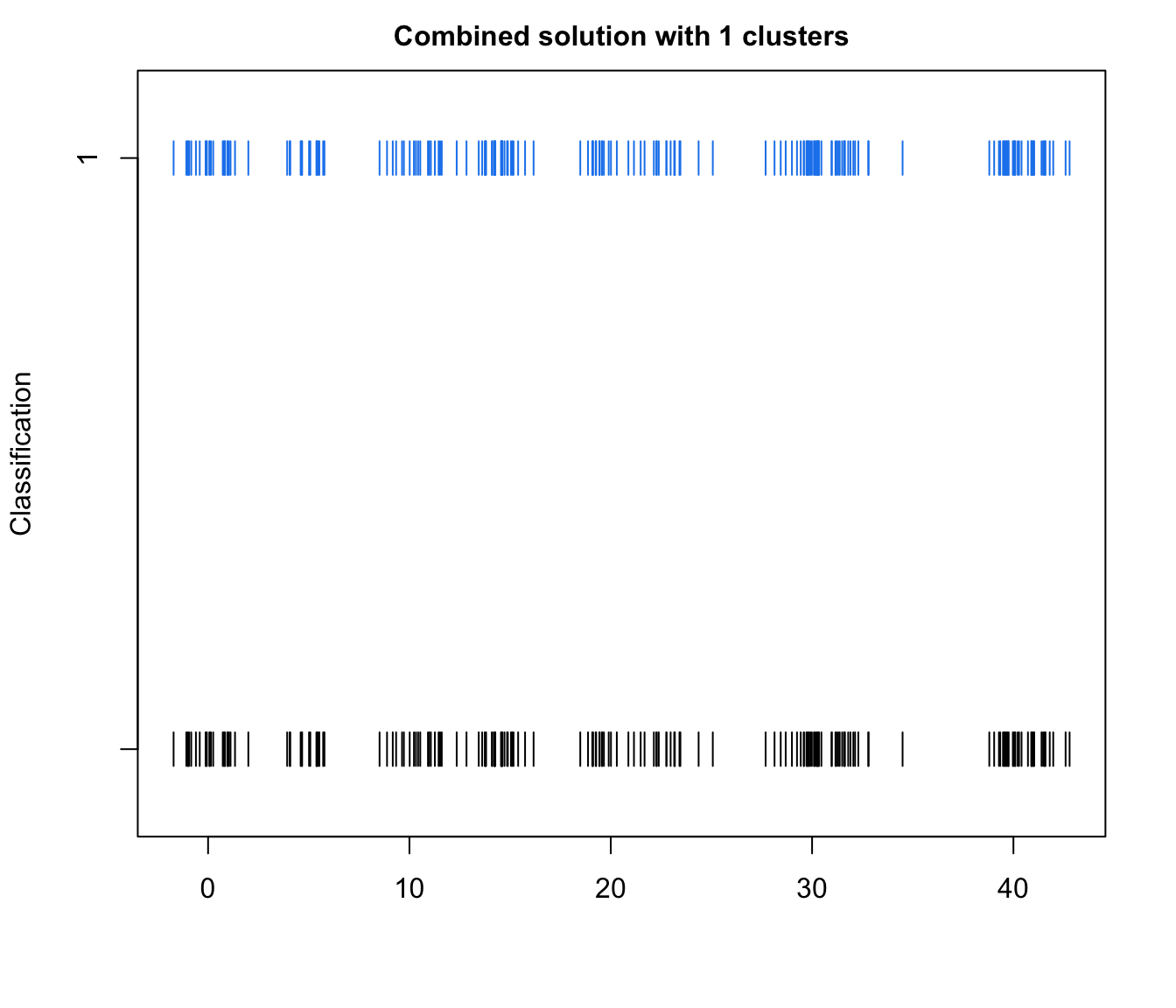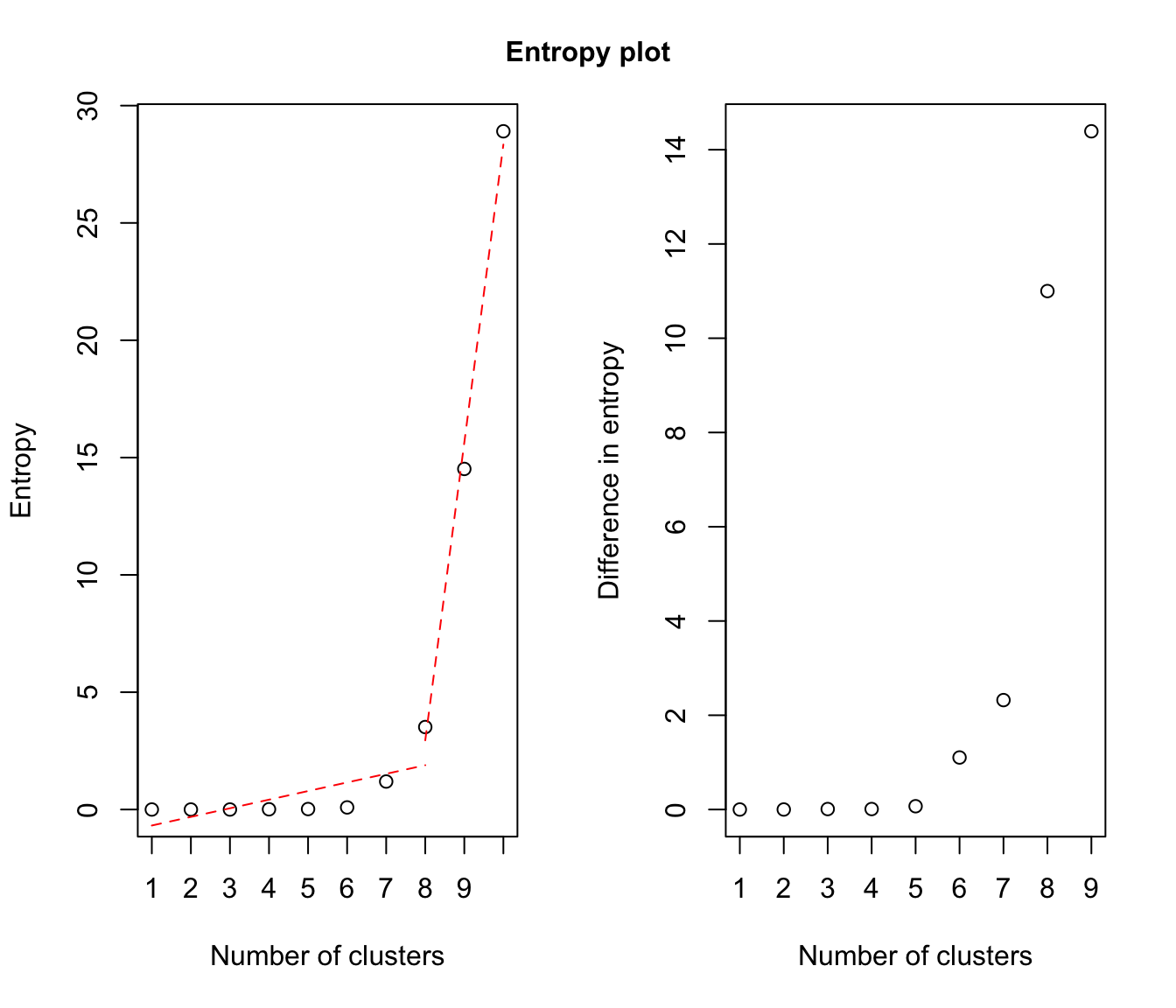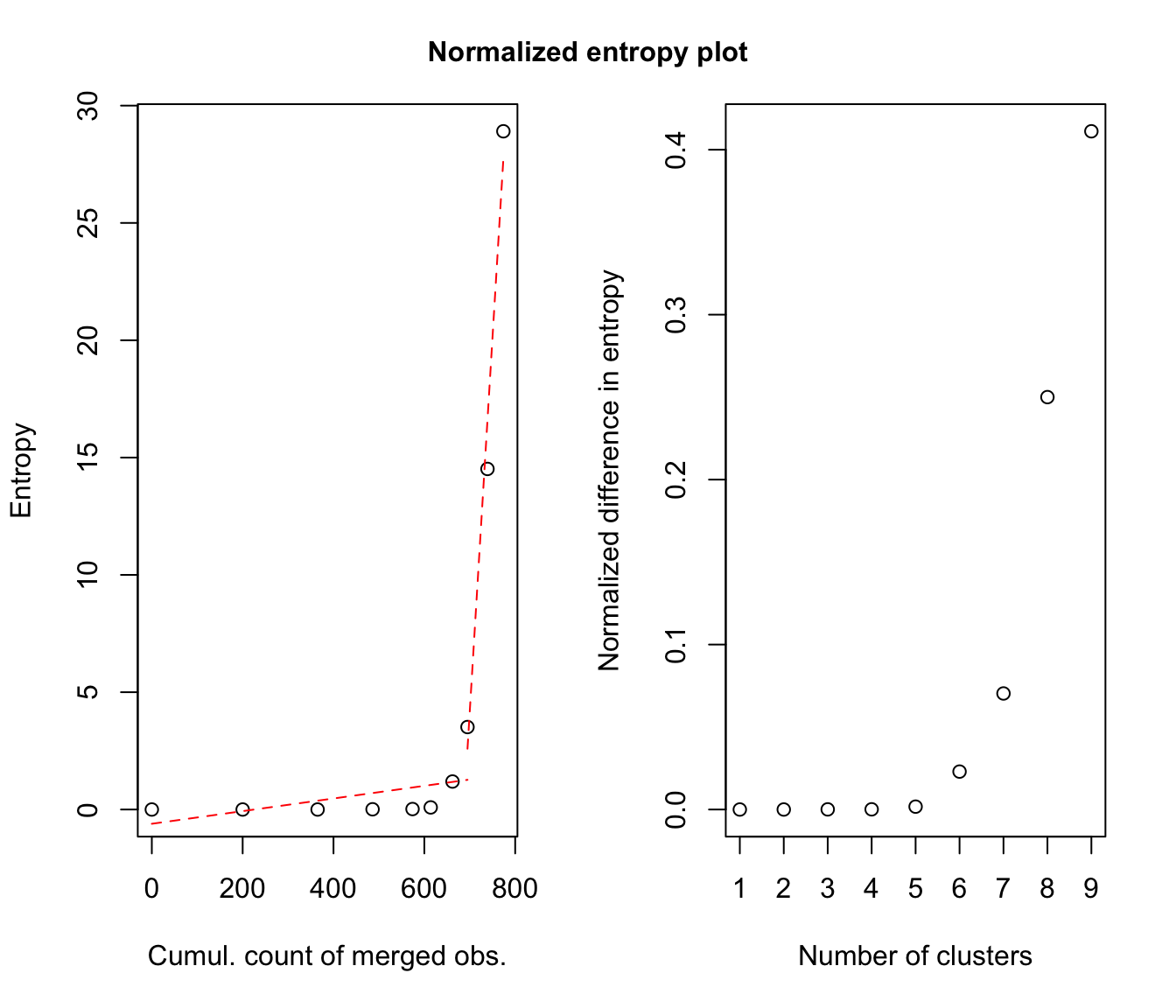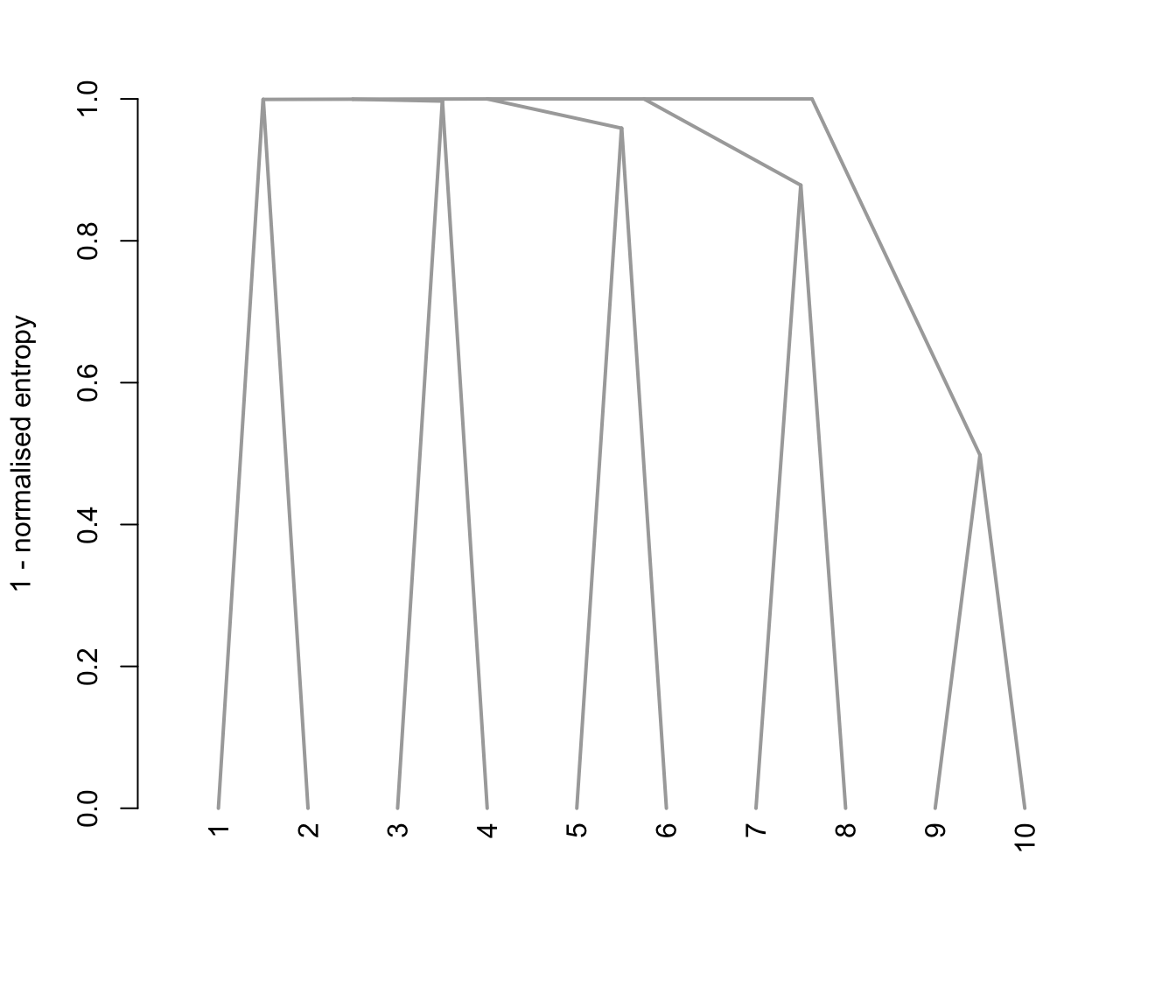## 2D Example
output <- clustCombi(data = ex4.1)

# plots the hierarchy of combined solutions, then some "entropy plots" which
# may help one to select the number of classes (please see the article cited
# in the references)
plot(output)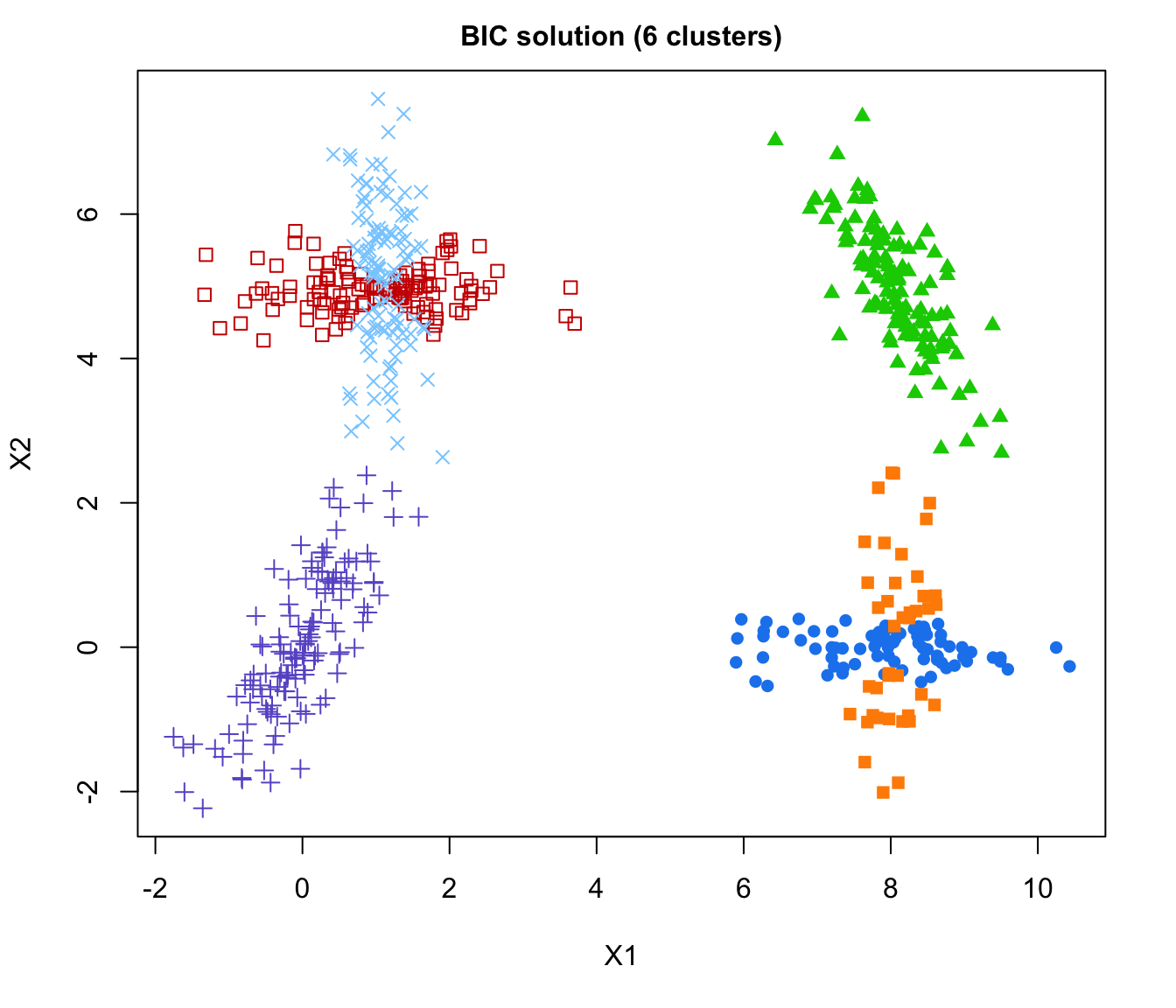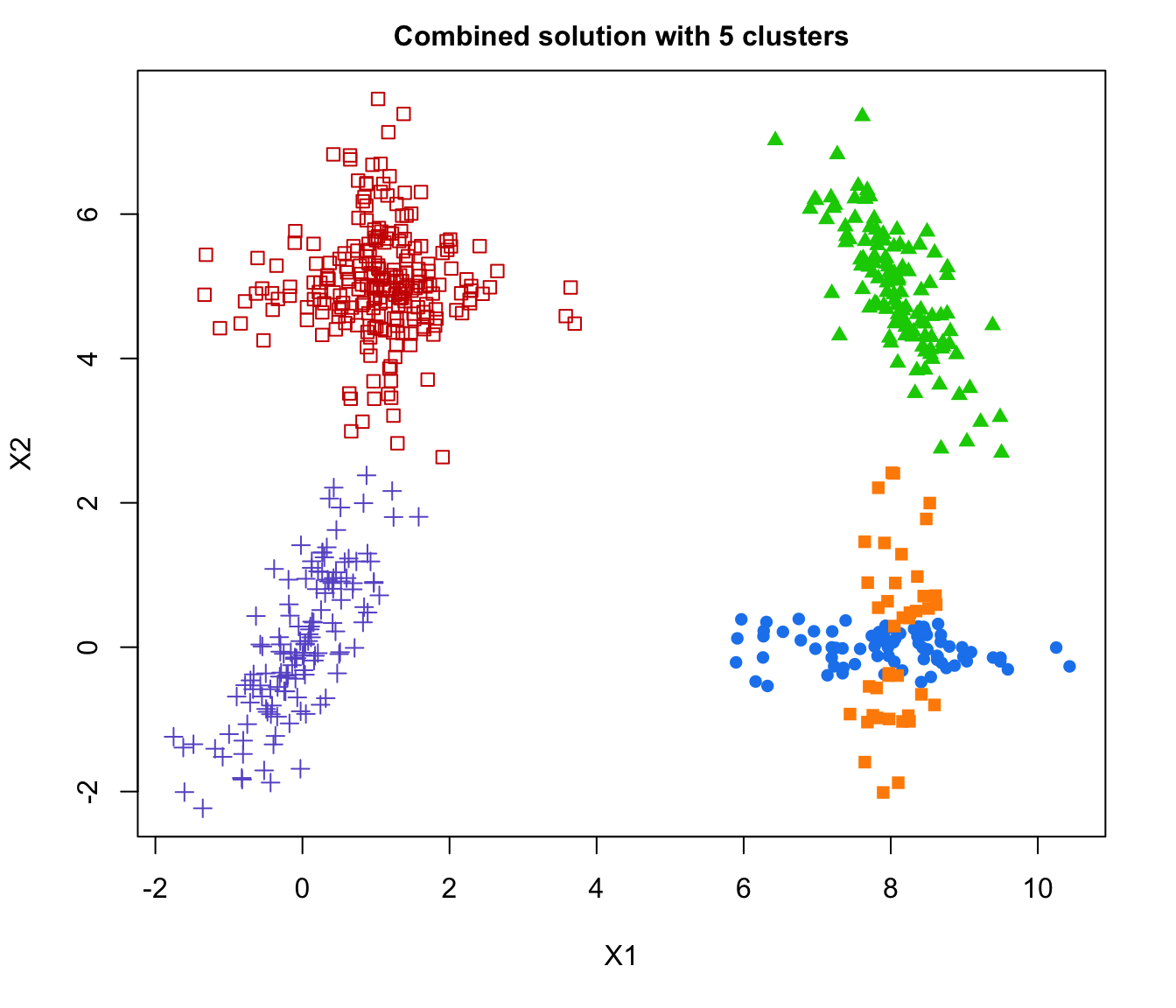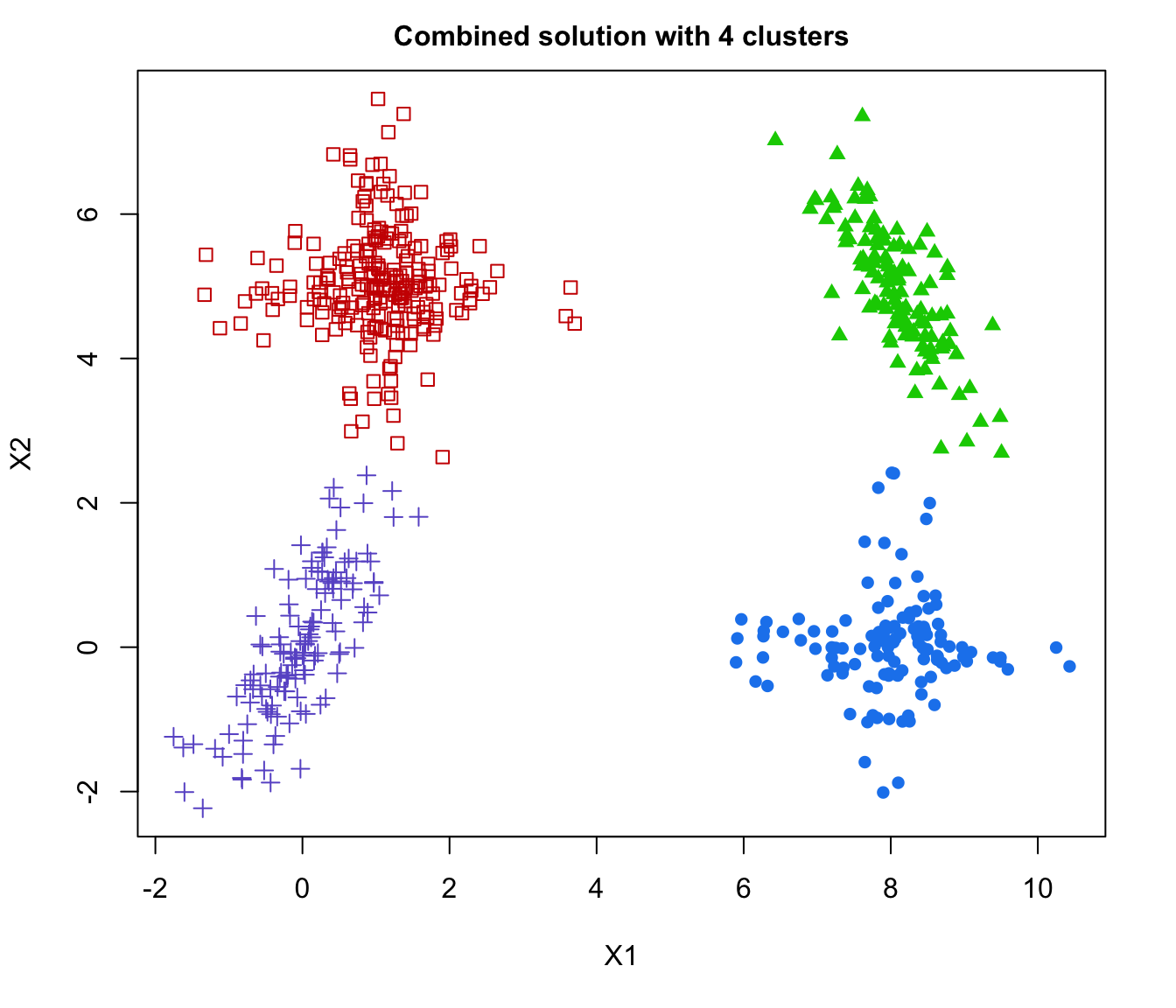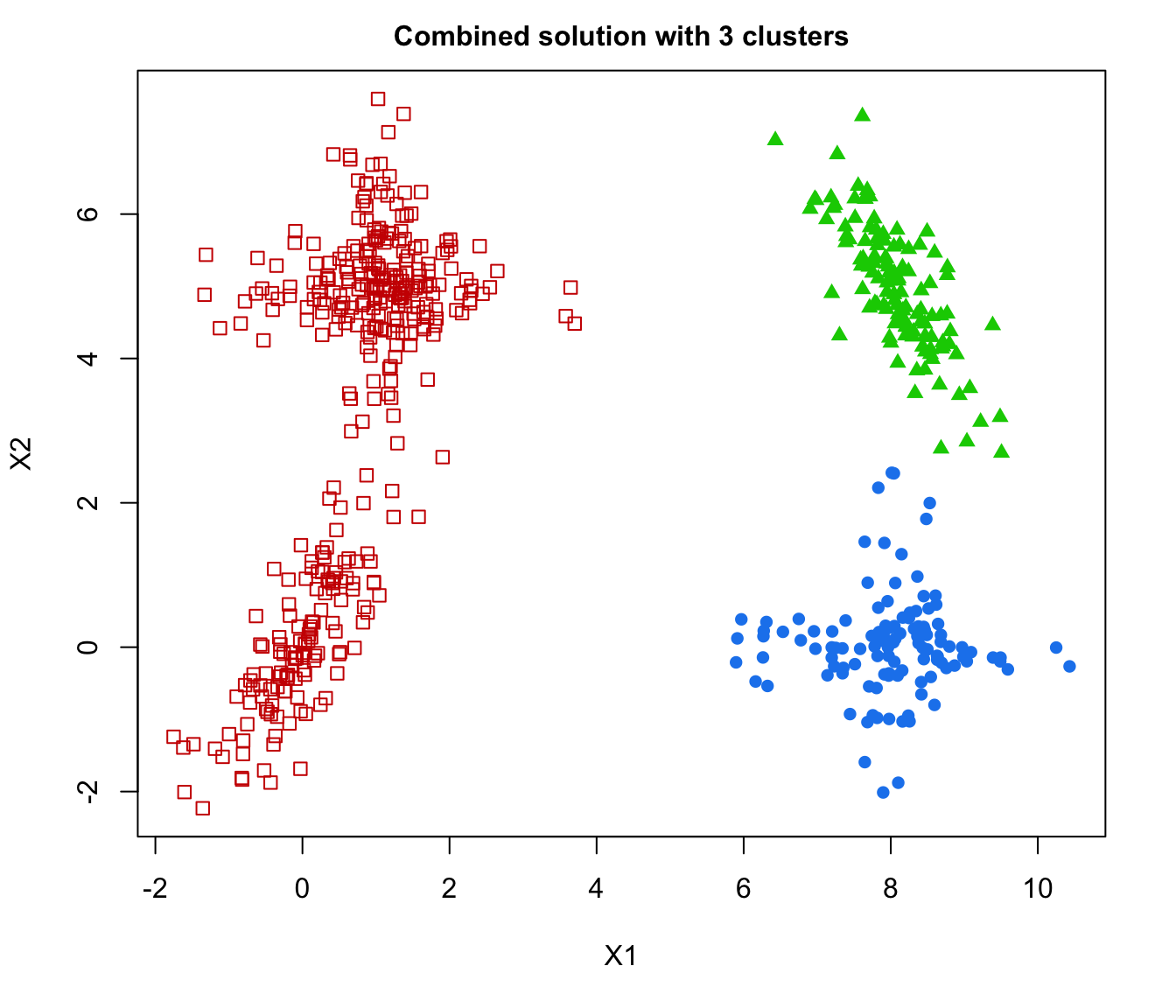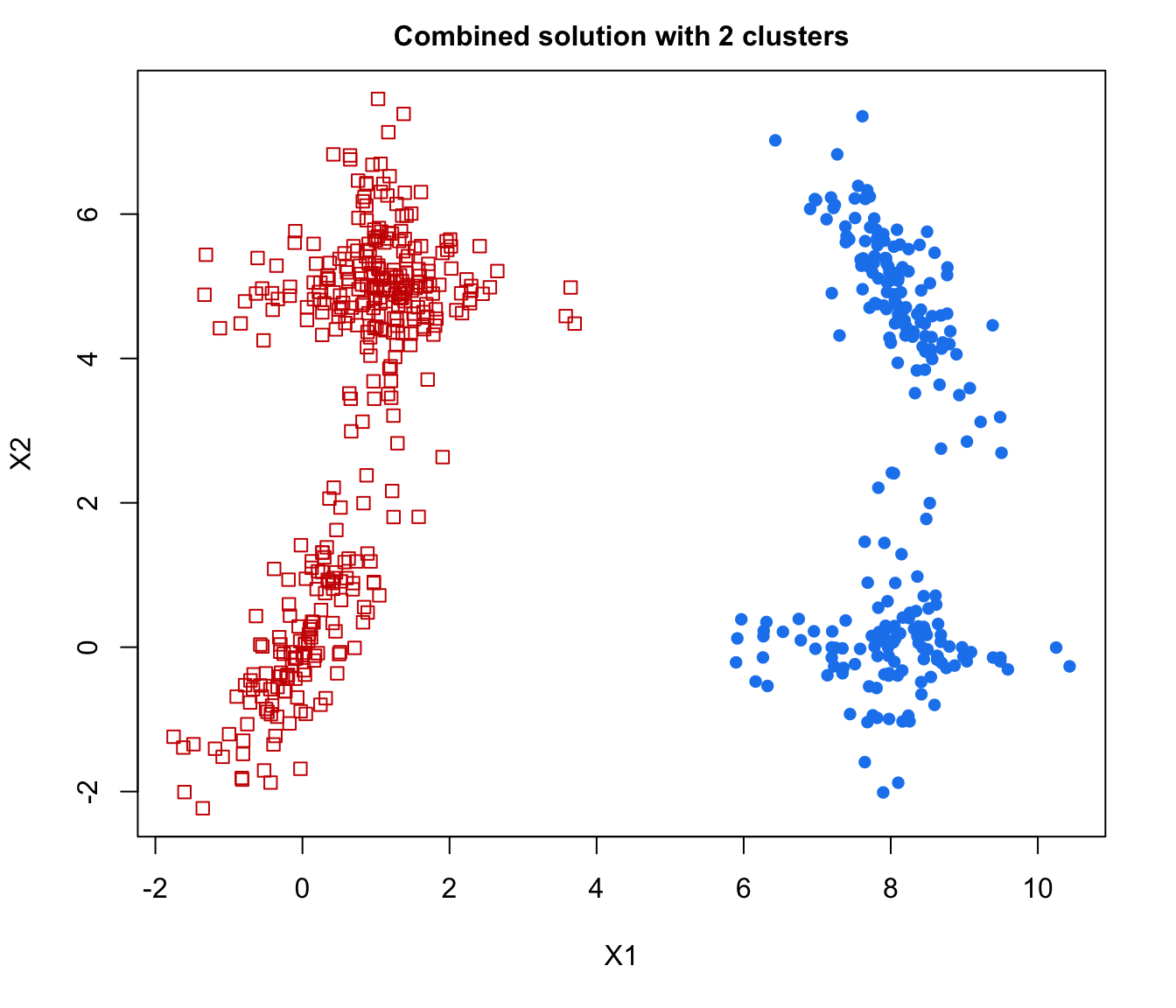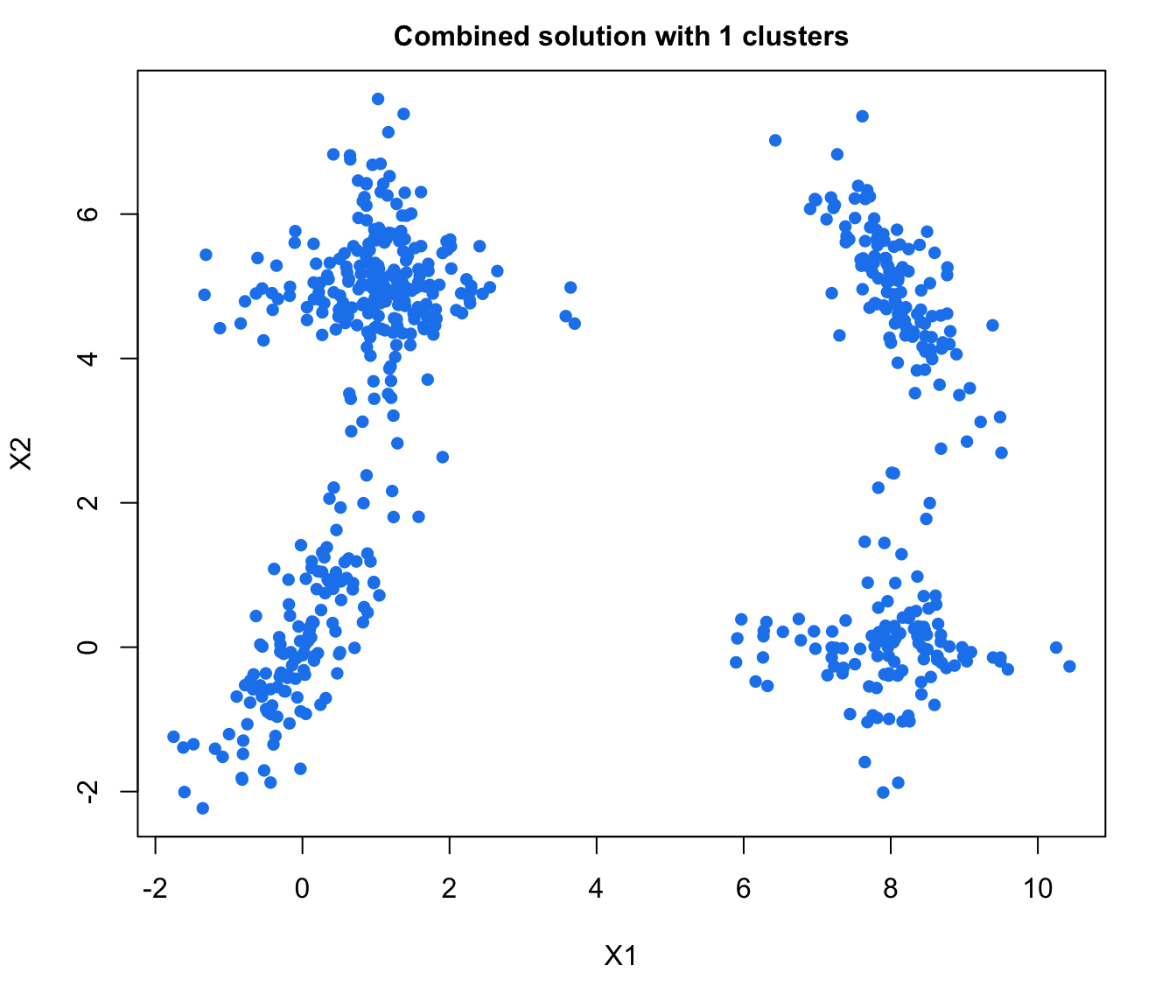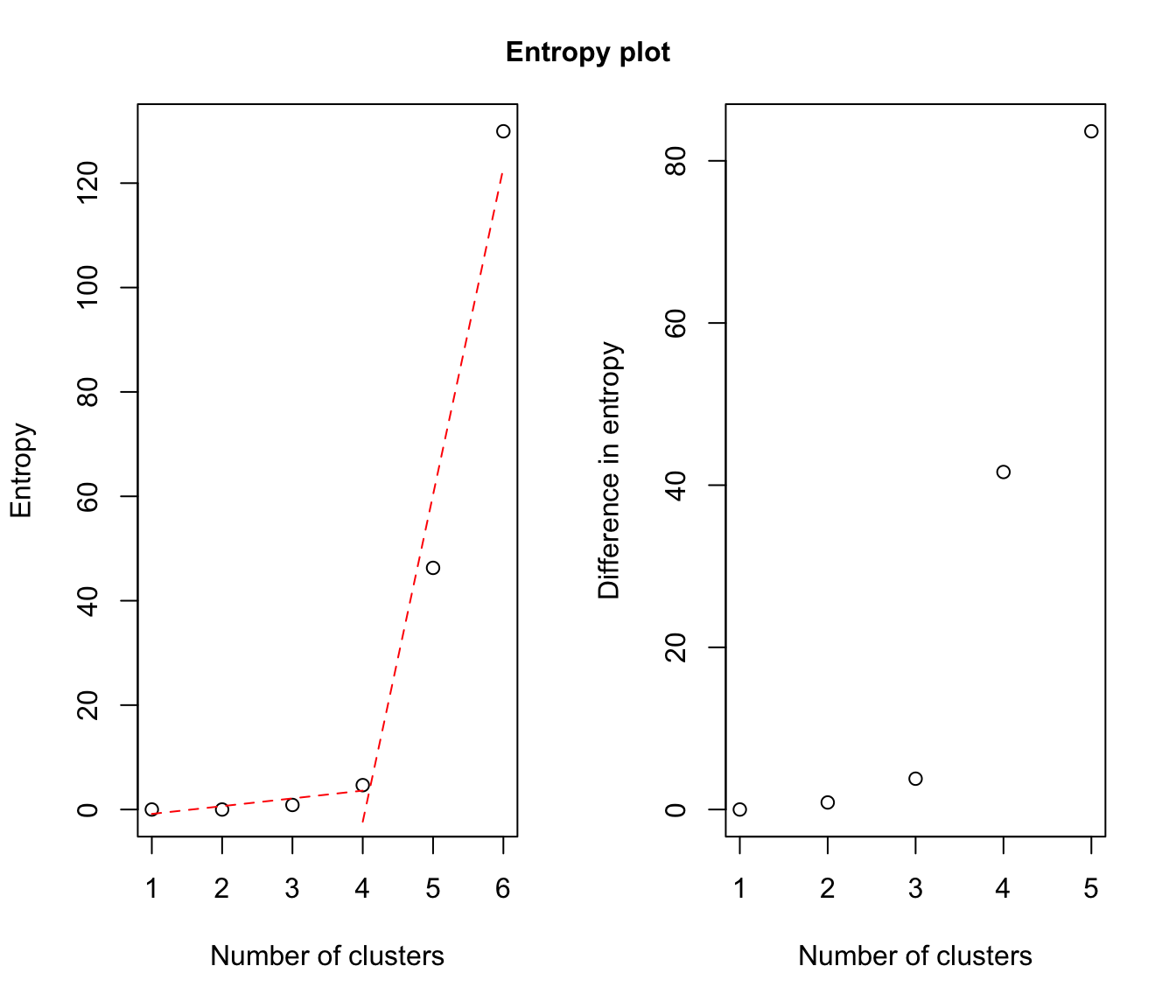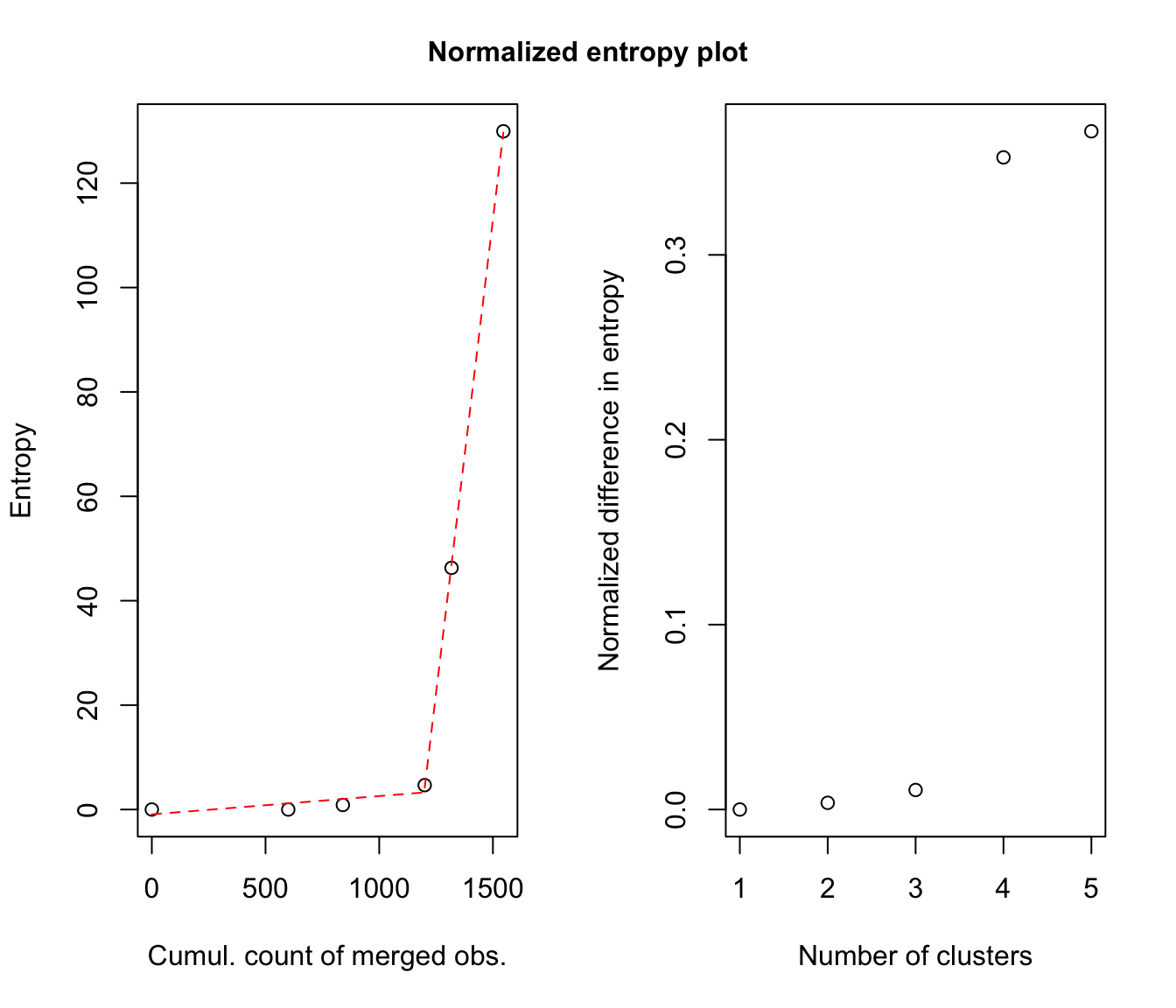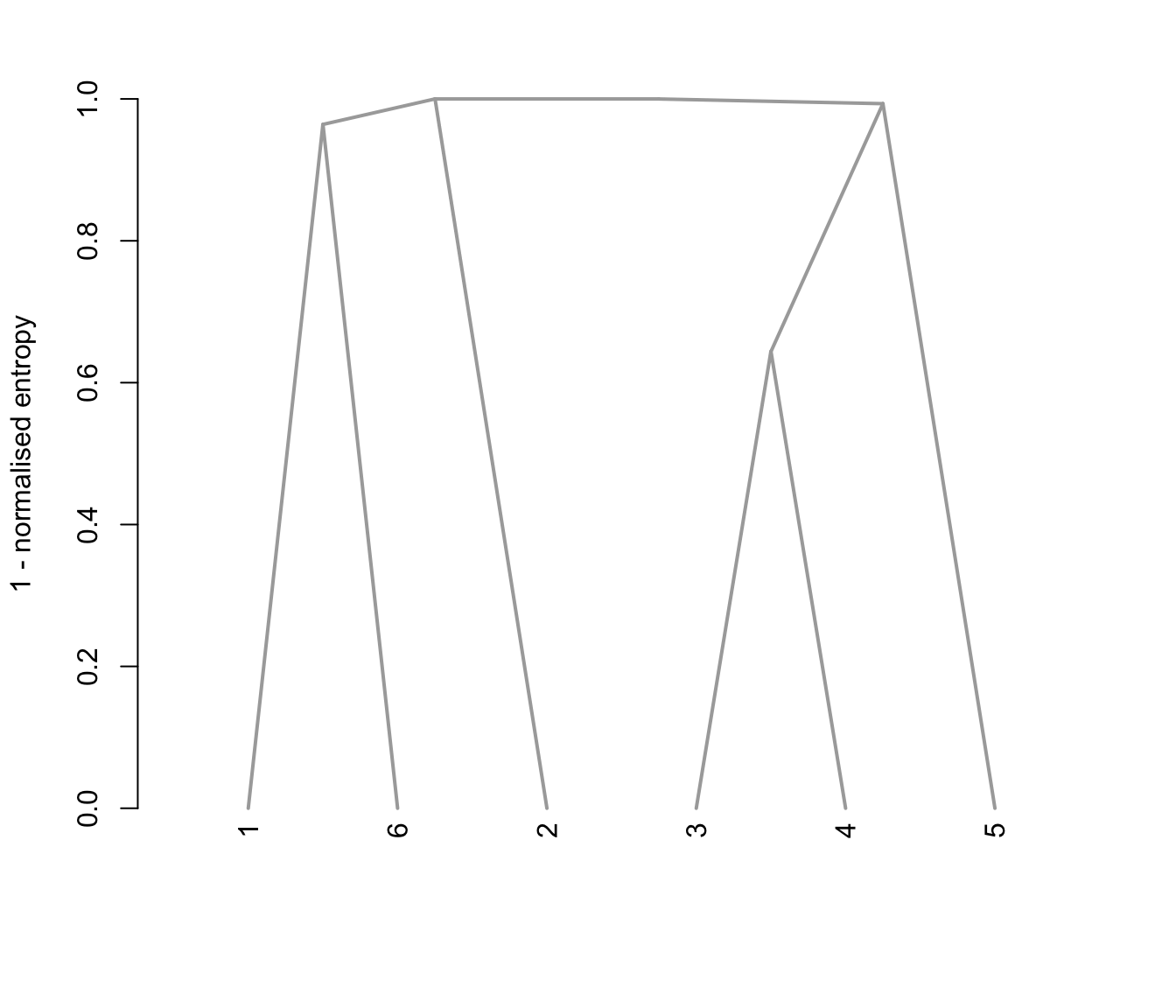## 3D Example
output <- clustCombi(data = ex4.4.2)

# plots the hierarchy of combined solutions, then some "entropy plots" which
# may help one to select the number of classes (please see the article cited
# in the references)
plot(output)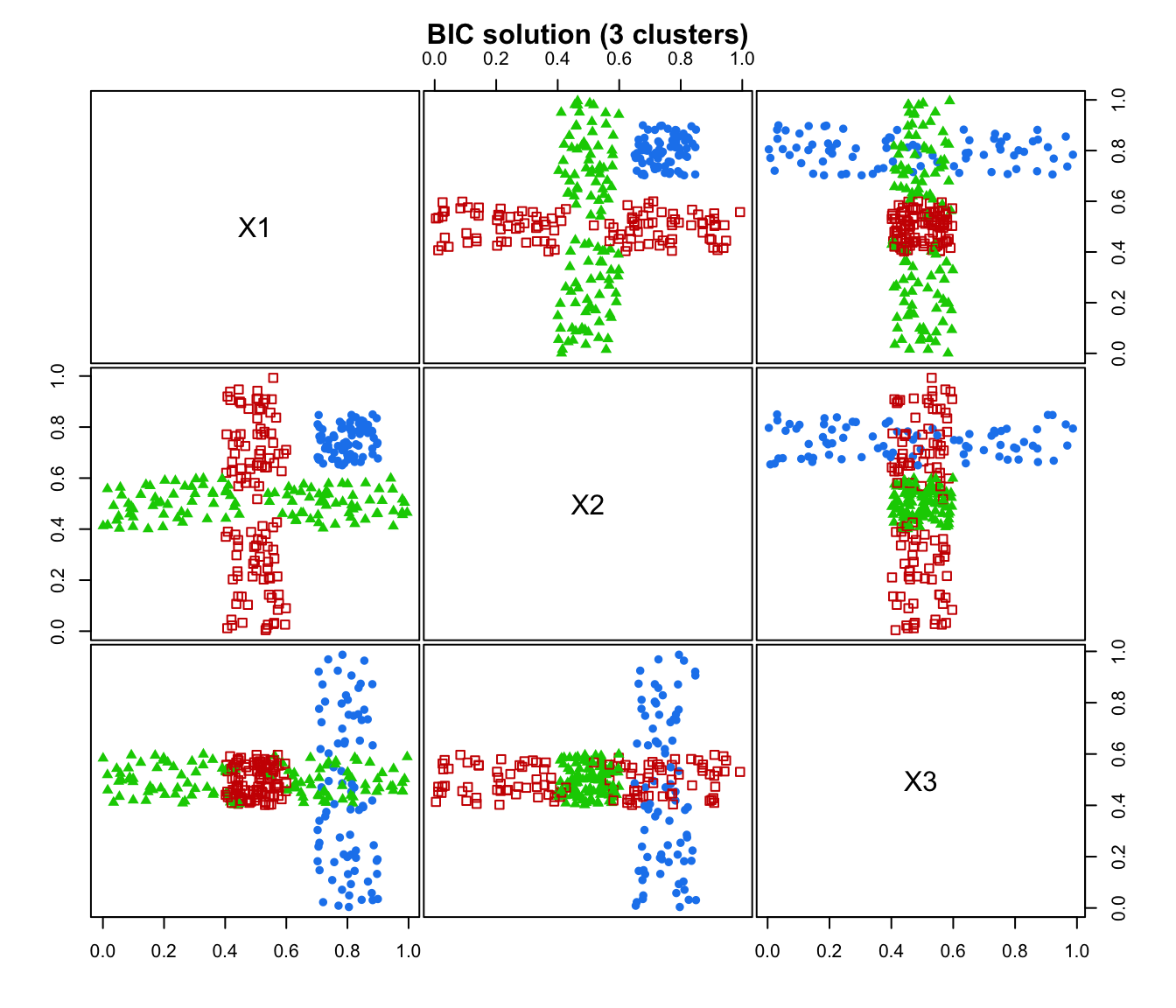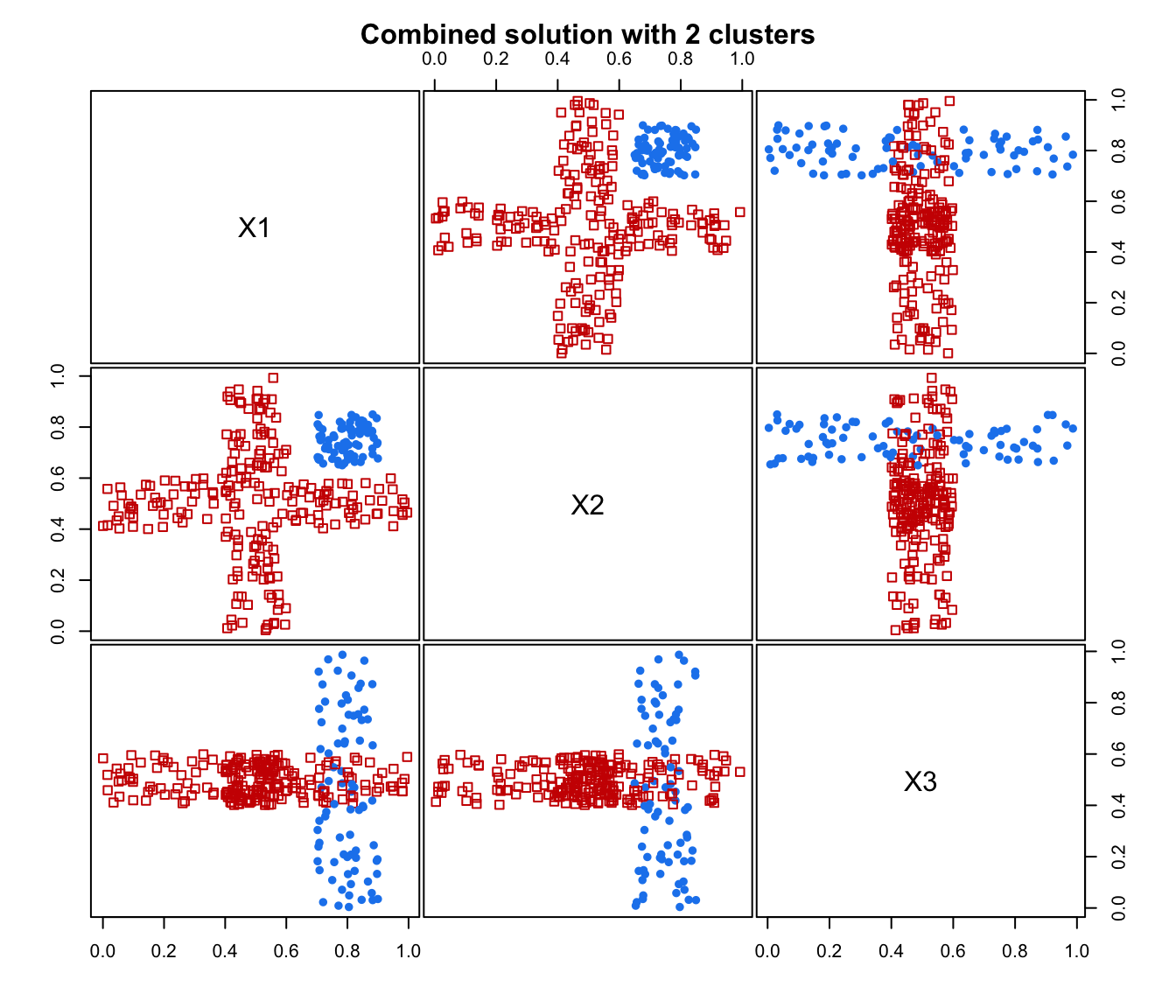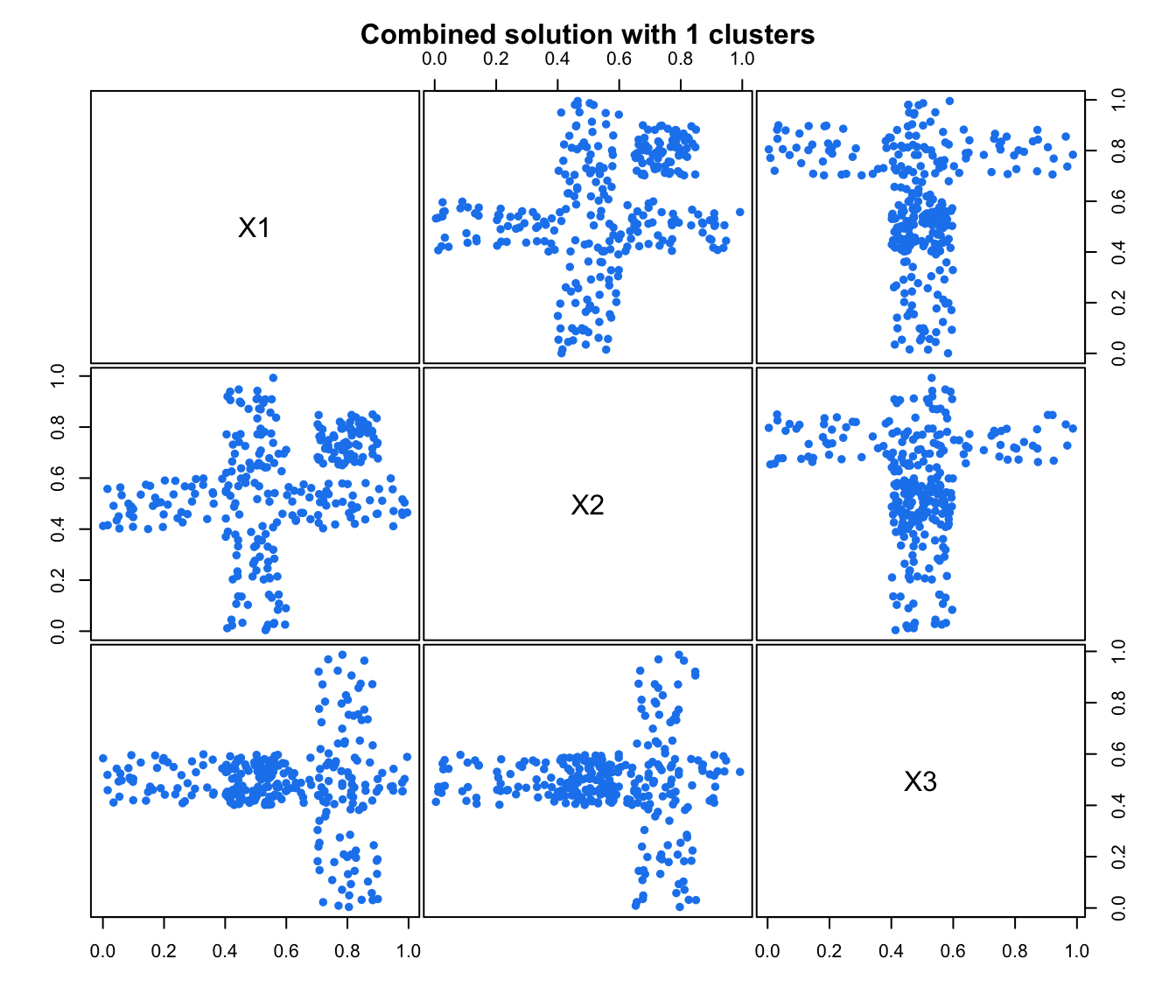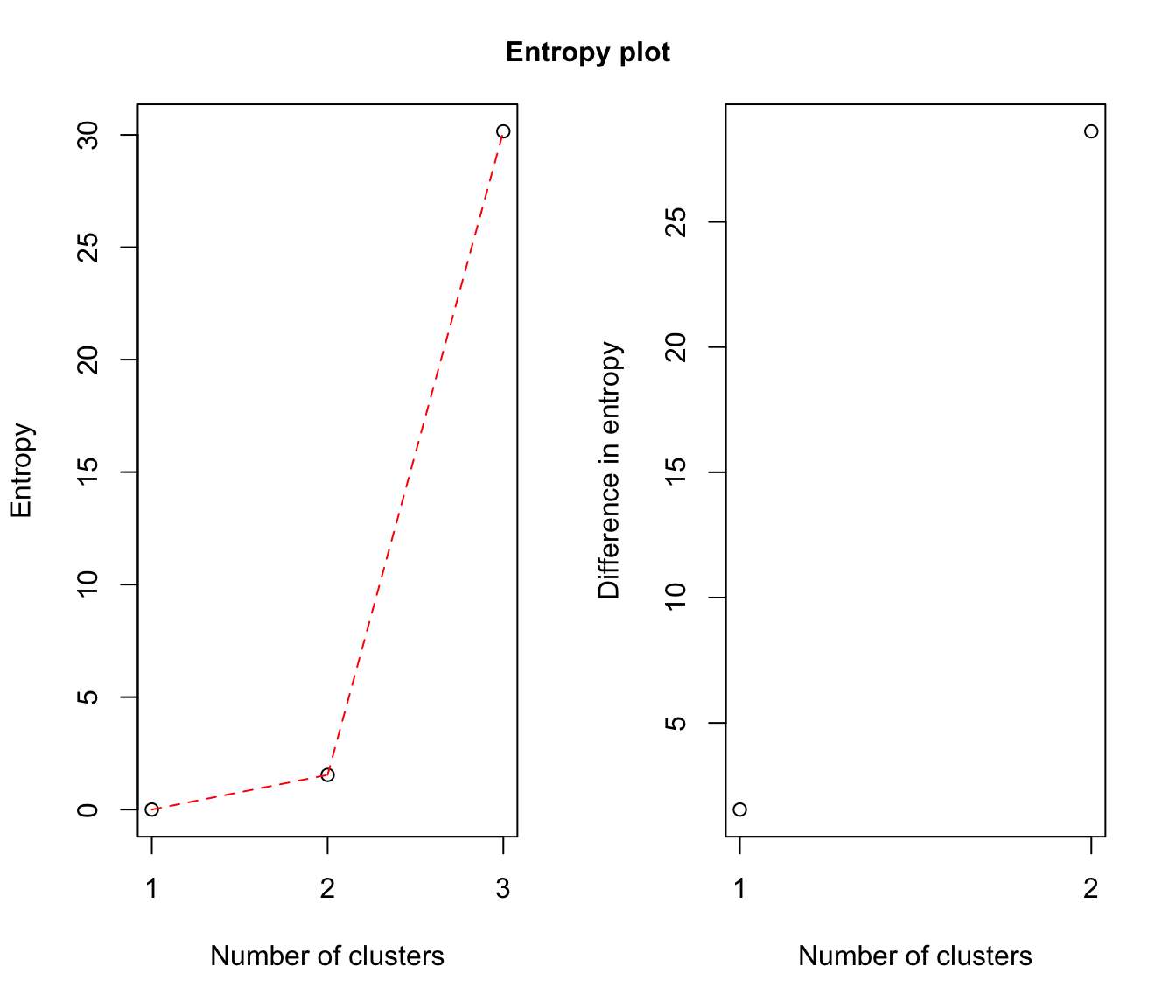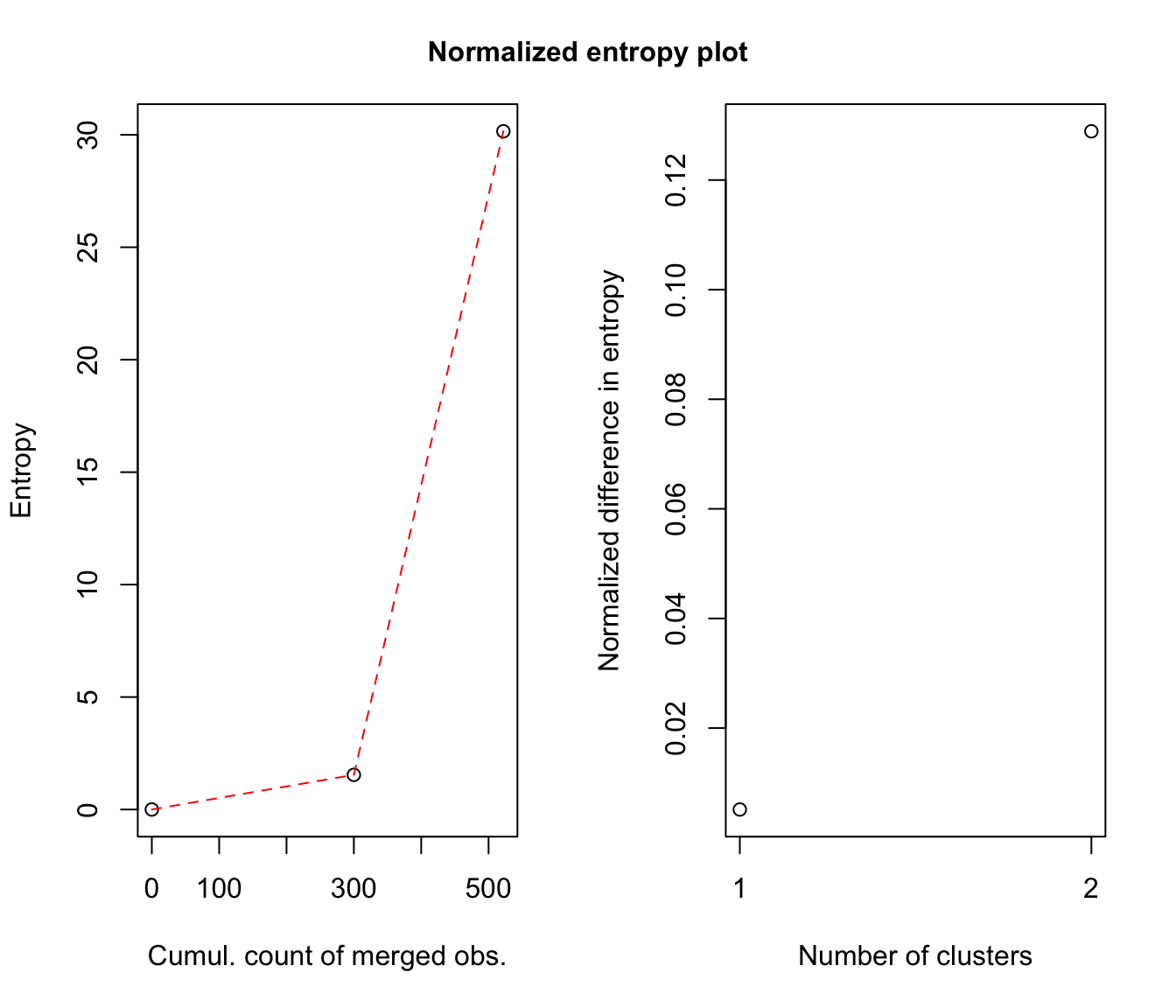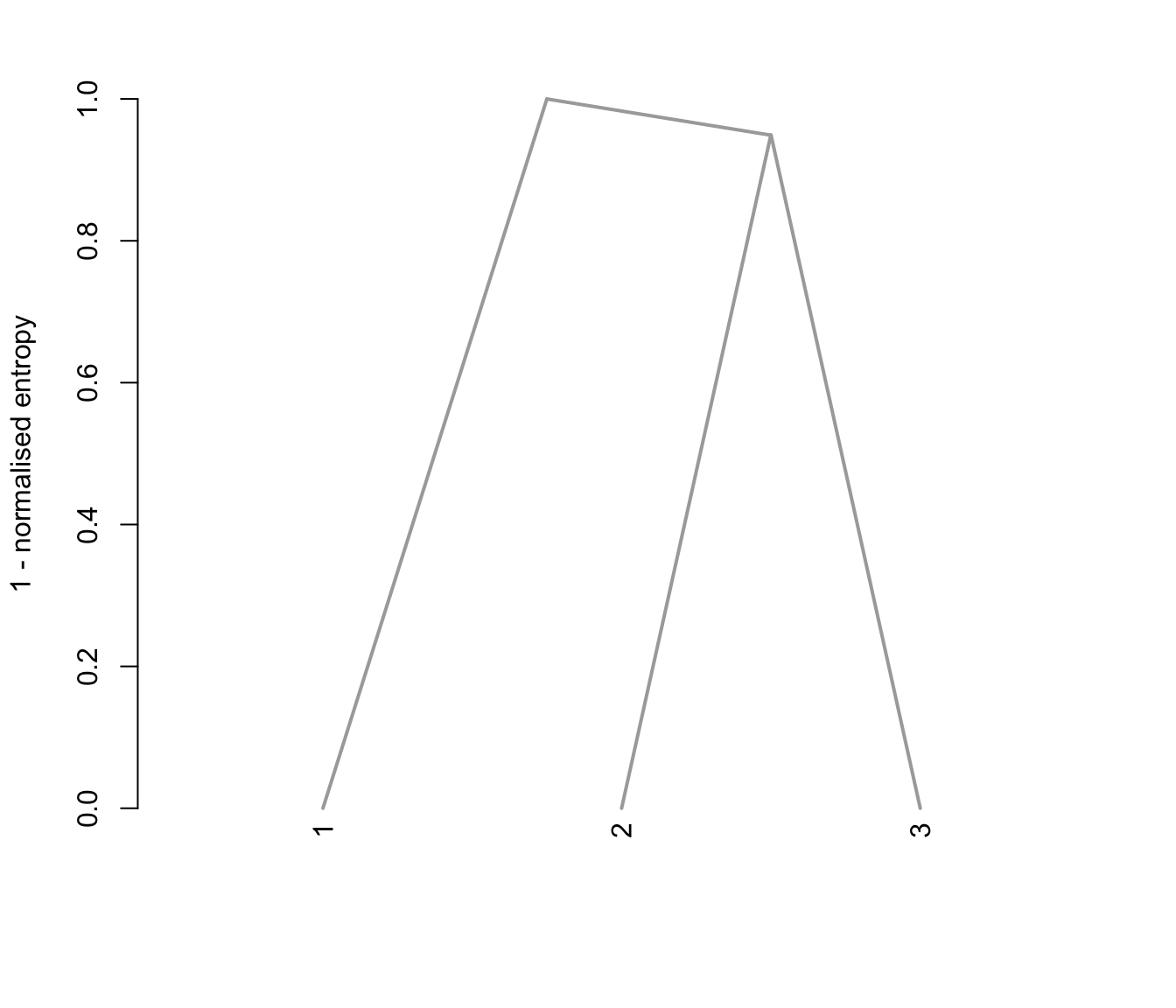# }